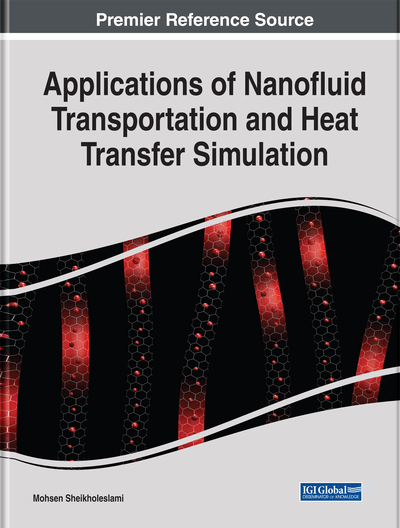# Space-Dependent Lorenz Forces Influence on Nanofluid Behavior

DOI: 10.4018/978-1-5225-7595-5.ch003

## Abstract

Magnetic nanofluid (Ferrofluid) is a magnetic colloidal suspension consisting of base liquid and magnetic nanoparticles with a size range of 5–15 nm in diameter coated with a surfactant layer. The effect of magnetic field on fluids is worth investigating due to its numerous applications in a wide range of fields. The study of interaction of the magnetic field or the electromagnetic field with fluids have been documented (e.g., among nuclear fusion, chemical engineering, medicine, and transformer cooling). The goal of nanofluid is to achieve the highest possible thermal properties at the smallest possible concentrations by uniform dispersion and stable suspension of nanoparticles in host fluids. In this chapter, the influence of external magnetic field on ferrofluid flow and heat transfer is investigated. Both effects of Ferrohydrodynamic (FHD) and Magnetohydrodynamic (MHD) have been taken in to account. So, the effects of Lorentz and Kelvin forces on hydrothermal behavior are examined.
Chapter Preview
Top

## 1. Effect Of Space Dependent Magnetic Field On Free Convection Of Fe3o4-Water Nanofluid

### 1.1. Problem Definition

The physical model along with the important geometrical parameters and the mesh of the enclosure used in the present CVFEM program are shown in Figure 1 (Sheikholeslami, 2014). The inner circular wall is maintained at constant hot temperature, the two horizontal walls are thermally isolated and other walls are maintained at constant cold temperature(>). To reach the shape of inner circular and outer rectangular boundary which consists of the right and top walls, a supper elliptic function can be used as follows;

(1)

For the expression of the magnetic field strength it can be considered that two magnetic sources at pointsand. The components of the magnetic field intensity () and the magnetic field strength () can be considered as (Sheikholeslami and Rashidi, 2015):

(2)(3)(4) wherethe magnetic field strength at the source (of the wire). The contours of the magnetic field strength are shown in Figure 2. In this study magnetic source is located atand

.

The flow is two-dimensional, laminar and incompressible. The magnetic Reynolds number is assumed to be small so that the induced magnetic field can be neglected compared to the applied magnetic field. Using the Boussinesq approximation, the governing equations of heat transfer and fluid flow for nanofluid can be obtained as follows:

(5)(6)(7)(8)

The termsandin (6) and (7), respectively, represent the components of magnetic force, per unit volume, and depend on the existence of the magnetic gradient on the corresponding x and y directions. These two terms are well known from FHD which is the so-called the Kelvin force. The term sandappearing in (6) and (7), respectively, represent the Lorentz force per unit volume towards the x and y directions and arise due to the electrical conductivity of the fluid. These two terms are known in MHD. The principles of MHD and FHD are combined in the mathematical model presented in Sheikholeslami and Ganji (2014) and the above mentioned terms arise together in the governing equations (6) and (7). The termin Equation (8) represents the thermal power per unit volume due to the magneto caloric effect. Also the termin (8) represents the Joule heating. For the variation of the magnetization, with the magnetic field intensityand temperature, the following relation is used Loukopoulos and Tzirtzilakis (2004):

(9) whereis a constant andis the Curie temperature.

(10)(11) whereis the solid volume fraction of nanoparticles. Thermal diffusivity of the nanofluid is(12) and the thermal expansion coefficient of the nanofluid can be determined as

(13)

The dynamic viscosity of the nanofluids is given is

(14)

The effective thermal conductivity of the nanofluid can be approximated by the Maxwell–Garnetts (MG) model as (Sheikholeslami, 2014):

(15) and the effective electrical conductivity of nanofluid was presented as below:

(16)

By introducing the following non-dimensional variables:

(17) where in Equation (17)and. Using the dimensionless parameters, the equations now become:(18)(19)(20)(21) where and are the Rayleigh number, Prandtl number, Hartmann number arising from MHD, temperature number, curie temperature number, Eckert number and Magnetic number arising from FHD the for the base fluid, respectively. The thermo physical properties of the nanofluid are given in Table 1 (Sheikholeslami and Ganji, 2014).

Table 1.
Thermo physical properties of water and nanoparticles

(Sheikholeslami and Ganji, 2014)

The stream function and vorticity are defined as:

(22)

The stream function satisfies the continuity Equation (18). The vorticity equation is obtained by eliminating the pressure between the two momentum equations, i.e. by taking y-derivative of Equation (20) and subtracting from it the x-derivative of Equation (19).

The boundary conditions as shown in Figure 1 are:on the inner circular boundaryon the outer wall boundaryon all solid boundaries(23)

The values of vorticity on the boundary of the enclosure can be obtained using the stream function formulation and the known velocity conditions during the iterative solution procedure.

The local Nusselt number of the nanofluid along the hot wall can be expressed as:

(24) where r is the radial direction. The average Nusselt number on the hot circular wall is evaluated as:

(25)

To estimate the enhancement of heat transfer between the case ofand the pure fluid (base fluid) case, the heat transfer enhancement is defined as:

(26)

## Complete Chapter List

Search this Book:
Reset